# Circuit Diagrams and Ohm's Law | Class 10 electricity Notes

## 6. Circuit Diagrams

• We already know that electric circuit is a continuous path consisting of cell (or a battery), a plug key, electrical component(s), and connecting wires.
• Electric circuits can be represented conveniently through a circuit diagram.
• A diagram which indicates how different components in a circuit have to be connected by using symbols for different electric components is called a circuit diagram.
• Table given below shows symbols used to represent some of the most commonly used electrical components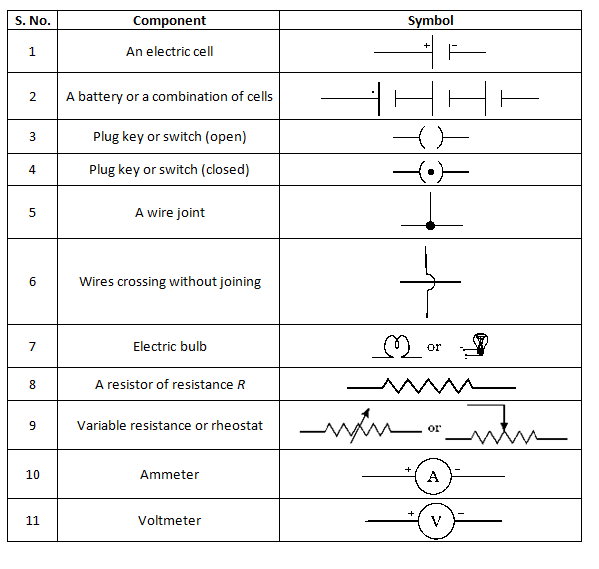## Ohm's Law

• Ohm's law is the relation between the potential difference applied to the ends of the conductor and current flowing through the conductor. This law was expressed by George Simon Ohm in 1826.
• Statement of Ohm's Law
If the physical state of the conductor (Temperature and mechanical strain etc.) remains unchanged, then current flowing through a conductor is always directly proportional to the potential difference across the two ends of the conductor
athematically
V ∝ I
or
V=IR
where constant of proportionality R is called the electric resistance or simply resistance of the conductor.
• Value of resistance depends upon the nature, dimension and physically dimensions of the conductor.
• From Ohm's Law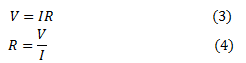Thus electric resistance is the ratio of potential difference across the two ends of conductor and amount of current flowing through the conductor.
• If a graph is drawn between the potential difference readings (V) and the corresponding current value (I), then the graph is found to be a straight line passing through the origin as shown below in the figure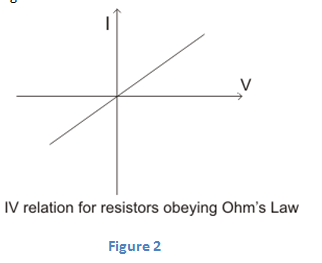• From graph we see that these two quantities V and I are directly proportional to one another.
• Also from this graph we see that current (I) increases with the potential difference (V) but their ratio V/I remain constant and this constant quantity as we have defined earlier is called the Resistance of the conductor.
• Electric resistance of a conductor is the obstruction offered by the conductor to the flow of the current through it.
• SI unit of resistance is Ohm (Ω) where 1 Ohm=1 volt/1 Ampere or 1Ω=1VA-1. Bigger units of resistance are Kilo-Ohm and Mega-Ohm
$1K\Omega = {10^3}\Omega$
$1M\Omega = {10^6}\Omega$
• The resistance of the conductor depends
1. on its length,
2. on its area of cross-section
3. on the nature of its material
• Resistance of a uniform metallic conductor is directly proportional to its length (l) and inversely proportional to the area of cross-section (A). That is,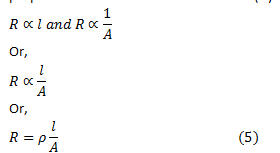Where ρ is the constant of proportionality and is called the electrical resistivity of the material of the conductor.
• The SI unit of resistivity is Ω m. It is a characteristic property of the material.
• The metals and alloys have very low resistivity in the range of 10-8 Ω m to 10-6 Ω m. They are good conductors of electricity.
• Insulators like rubber and glass have resistivity of the order of 1012 to 1017 Ω m.
• Both the resistance and resistivity of a material vary with temperature.

### Ohmic and Non-Ohmic resistors (or devices)

From above figure 2 we can see that straight line graph means that ratio V/I is constant . This constant ratio is called resistance R of the conductor. Resistance may be ohmic or non-ohmic.
(a) Resistors (or devices) for which potential difference and current graph is a straight line are called ohmic resistors. Their resistance remains same throughout their operation.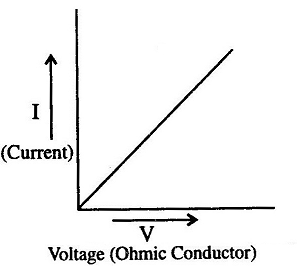Examples are metallic conductors.
(b) Resistors (or devices) for which potential difference-current graph is not a straight line are called non-ohmic resistors.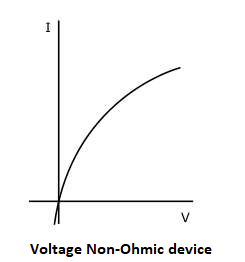Examples are liquid electrolytes, diodes etc.

### Concept Map of Ohm's Law### Solved Questions on Ohm's Law Class 10

Question 1. What causes resistance in a conductor?
Answer. When electrons move from one end of the conductor to the other end , then because of the potential difference they collide with the electrons, atoms and ions present in the conductor. Due to these collisions movement of the electrons gets restricted through the conductor. Thus “resistance is the property of the conductor due to which it opposes the flow of charge”.
Solved Example 1. Following graph was plotted between V and I values.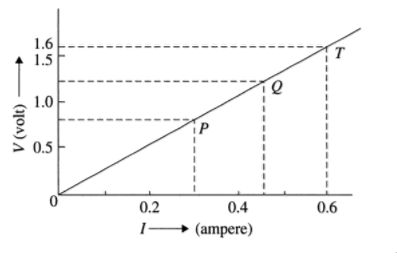What would be the values of VI/ ratios when the potential difference is 0.8V, 1.2V and 1.6V respectively? What conclusion do you draw from these values?
Solution
Hint: Choose convenient scales for X-axis and Y-axis (X-axis, 1 cm= 0.1 A, Y-axis, 1 cm= 0.5 V) and mark the current bid potential difference values as shown. Draw horizontal lines corresponding to the potential difference values of 0.8V, 1.2V and 1.6V; which meet the graph at P. Q and T respectively. From P. Q and T draw vertical lines to the X-axis and get the respective current values. Tabulate as given below and find the values of V/I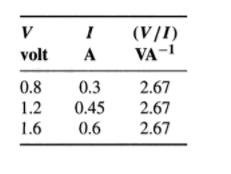From the nature of the graph (which is a straight line) and above table we can conclude that ￼ is same at every point. We find that V/I is same and found to be a constant. The wire obeys ohm's law and its resistance R = 2.67 ohm.
Solved Example 2. Electrical resistivity's of some substances at 20°C are given below: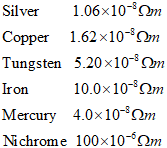Answer the following questions using above data:
(a) Among silver and copper, which one is a better conductor? Why?
(b) Which material would you advise to be used in electrical heating devices and why?
Answer. (a) Silver is better conductor as its resistivity is less then that of copper.
(b) Nichrome, as it has a greater value of resistivity as compared to the others.
Solved Example 3.  A simple electric circuit has 24 V battery and a resistor of 60 ohm. What will be the current in the circuit. The resistance of the connecting wire is negligible.
Solution. Here Voltage (potential difference)=24 V
Circuit resistance , R= 60 Ohm
Current I=? To be calculated
From Ohm’s Law
$R = \frac{V}{I}$
Or we have
$I = \frac{V}{R}$
Putting the values we get
$I = \frac{{24V}}{{60\Omega }} = 0.4A$
The current in the circuit will be 0.4A.

Given below are the links of some of the reference books for class 10 Science.

You can use above books for extra knowledge and practicing different questions.

### Practice Question

Question 1 Which among the following is not a base?
A) NaOH
B) $NH_4OH$
C) $C_2H_5OH$
D) KOH
Question 2 What is the minimum resistance which can be made using five resistors each of 1/2 Ohm?
A) 1/10 Ohm
B) 1/25 ohm
C) 10 ohm
D) 2 ohm
Question 3 Which of the following statement is incorrect? ?
A) For every hormone there is a gene
B) For production of every enzyme there is a gene
C) For every molecule of fat there is a gene
D) For every protein there is a gene

Note to our visitors :-

Thanks for visiting our website.